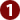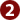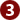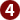STUDY
AND
EXAM
.COM

# Preparation Guidelines for GRE General Test

GRE General test assesses the general academic proficiency of students coming from varying educational backgrounds. Every year thousands of students appear in GRE general to pursue higher education or to seek scholarship in the top ranking educational institution of the world. Despite hard work, some students fail to secure the desired score in GRE test because they do not make preparation for GRE in proper lines. This article encompasses all the guidelines for preparation which ensure your success in GRE General test.

The guidelines and tips for GRE test are divided into following:

1. Understanding the Test Format
2. Preparation for Verbal Reasoning
3. Preparation for Quantitative Reasoning
4. Preparation for Analytical writing## Understanding the Test Format

The first step towards your preparation for GRE test is to understand the format of the test. You should know the correct sequence of parts of the test, the number of question in each section, nature of questions, and time for each section. Familiarize yourself with the structure of test. It enhances your confidence level during the test as well as helps you complete the test in time.

GRE general is a computer-based test. The total time of test is 3 hours and 45 minutes which also include 10 minutes-break between the sections. The format of test reflecting three major parts of test and section in correct sequence is given below:

ANALYTICAL WRITING
Task-1 Analysis of an Issue 30 Minutes
Task-2 Analysis of an argument 30 Minutes
VERBAL REASONING
Section-1 20 MCQs 30 Minutes
Section-2 20 MCQs 30 Minutes
QUANTITATIVE REASONING
Section-1 20 MCQs 35 Minutes
Section-2 20 MCQs 35 Minute

Unscored Questions: A few unscored questions are intentionally included in the test (in verbal & quantitative part only) which carry no marks and have no impact on your overall marks. These questions can appear in any sequence in the question and cannot be identified. Hence, you will treat every question as it has valid marks.

The purpose of adding unscored questions to the test is that it helps ETS to refine their MCQs bank for future tests. For this reason, ETS may add a specific section at the end in some tests with the title of “unscored section or research question”. In such a case the unscored questions are identifiable though they carry no marks.## Preparation for Verbal Reasoning

The verbal reasoning part is designed to assess your proficiency in the English language with regard to vocabulary and their correct utility according to grammar rules. This part has following three types of questions:

• Sentence equivalence               (6 questions per section)
• Text completion                             (4 questions per section)
• Reading comprehension           (5 questions per section)

The new GRE General test has no Antonyms and Analogies question. The guidelines for preparation are as follows:

1. Sentence equivalence question has one blank. You have to select two words from the given options which (if either of the two is used in the blank) the sentence must give the same meaning. Remember! The question instructions state to select two words, using either of which, the sentence must give a similar meaning. It means that these two words must be near synonyms. Hence, you should focus on choosing two words having a similar meaning.
2. A few questions may also have 3 or 4 synonyms (for a single word) in the given options. In such a case, you have to choose only the 2 synonyms which, apart from having similar meaning, also make the correct grammatical structure of the sentence.
3. Text completion question is a short paragraph (of 1 to 5 sentences). Each question has a varying number of blanks (i.e. one, two or three blanks).  For each blank, a separate set of five choices (word or phrases) will be given. It means a question having one blank will have only one set of five choices while question having 2 blanks will have 2 separate sets of five choices. You have to choose the correct choice which makes the sentence grammatically correct as well as verbally correct.
4. In reading comprehension, you will be given a piece of literature of 4 to 5 paragraphs. These paragraphs are usually extracted from books, magazine, publications, research papers, description from disciplines such as science, arts, politics humanities or routine happenings.
5. You have to answer the questions given at the end. Each question has choices to choose from but one type of question added in the GRE test is that you have to click on a sentence within the paragraph to answer it.
6. For the verbal part, you should focus on two areas: Vocabulary of English language and basics of English Grammar. Having a good vocabulary is very much important, as the verbal part was once known as Vocabulary test. To enhance your vocabulary, you should learn the ‘high-frequency words’ of English. These are the sophisticated words highly used in written English and commonly asked in such test. If you have time for preparation, learning these words can double your GRE score as they help you in different ways in the test.
7. On the second number, learning the basics of English Grammar is vital to your success in GRE test. Have some time to understand the basic concept of English Grammar such as ‘subject-verb agreement, dangling modifiers, redundancy and formation of clause and phrase’. Most of the question assess your proficiency in terms of your knowledge about the application of such grammar rules.## Preparation for Quantitative Reasoning

The quantitative part assesses your mathematical and statistical skills acquired at high school. The quantitative question pertains to following areas:

• Simple numerical quantification
• Percentages
• Ratio and proportion
• Basic algebra
• Basic geometry
• Data interpretation

The following guidelines should be followed for preparation:

1. These questions involve simple arithmetics as these are designed for all students coming from different educational background. Most of the questions are based on easy arithmetics such as addition, subtraction, division or multiplication, but the questions involve arithmetic tricks.
2. Most of the questions require short formulas to be solved quickly. Learn these formulas for common question. For instance, a common question asked is ‘what is the sum of natural numbers from 1 to 200?’. Adding all the number from 1 to 200 takes hours. But it has a simple formula:
3. Sum of natural digits = total number of digits x(first digit + last digit)/2
Sum of natural digits =   200(1+200)/2   = 20100 Ans

4. Learn the principles of calculating percentage with regard to changing scenarios. For instance, you may be asked an easy question that the population of an area increased from 10000 to 12000 in a year, what is the increase in population in percentage?
5. Learn the basics of ration and proportion in both direct relation between the values (i.e. if 7 person make 65 machines in 3 hours, how many machines will be made by 13 person in 3 hours?) as well as inverse relation between the values (i.e. If a specific amount of food is enough for 15 persons for 20 days, for how many days will be the same amount of food enough for 25 persons?)
6. Learn the basics of Geometry i.e. formulas of areas of square, rectangle, triangle and circle. Similarly learn the formulas of volume of cube, cylinder and circular bodies and formulas of circumference of circle. Remember, some questions will have clear application of these formulas but in some question the application of these formulas would require a bit brain storming. For instance, you may be asked to calculate area of an unusual shape. Such shapes are usually combination of many shapes e.g. a rectangle, a triangle and a semi-circle. Hence, you are required to calculate area of each shape within the unusual figure and add them to get total area of the unusual figure.
7. You must learn the application of Pythagorean Theorem as it is very favorite area of test makers. Using this theorem we calculate length of one side of triangle when the lengths of other two sides are given. For instance, a commonly asked question is you are given length of a wall and floor and you are asked to find length of ladder to reach to the top of the wall. Question like this and other present triangular scenario which are solved with the help of Pythagorean Theorem.## Preparation for Analytical Writing

Analytical writing part assesses your literary skills in terms of usage of wide range of vocabulary and grammatical accuracy in essay writing. This has two task of essay writing as follows:

• Essay writing on an Issue (one to be selected from given two topics).
• Essay writing on an argument

Essay writing on an Issue: You will be a statement expressing a debatable issue. It can be a common issue which is usually debated in various academic or non-academic circles. Some people have a positive attitude towards it and some have a negative attitude towards it. Here are some examples: Is the use of internet good for students? Should abortion be legalized? Whether the modernization is leading toward social prosperity or social frustration? These statements can be in a sentence form or in a topic form. You will be given instruction to explain your stance on the issue.  Remember the following points:

1. Spend 3 to 5 minutes to map out the positive and negative aspects of the issue.
2. Your stance should be clear on the issue.
3. You should support your stance with logical ideas.
4. Given examples to support your ideas.
5. Giving some statistical or verbal facts in the essay will make your essay look appealing.
6. In some topics, it is easy to choose one side but some topics have both sides worth explaining in the essay e.g. Pros and Cons of something. Your essay should majorly focus on your chosen stance but should also have some space for the opposing stance if required.
7. Use a wide range of words instead using same words repeatedly.
8. Learn the correct structure of writing essay and paragraphs. An essay has three parts: 1. Introduction, 2. Body, 3. Conclusion. Each paragraph has a first introductory sentence and the next sentences of the same paragraph elaborate the introductory sentence.
Essay writing on an argument:  You will be given an argument expressing a logic. The logic can be a cause-effect relationship, a judgment or observation, an assumption, a thought provoking idea and so on. You have to understand the logic of the argument and elaborate it further in your words. Remember, the elaboration should not include much of your personal views; rather the elaboration should be strictly based on the information given in the argument. For instance, the sale of a mobile phone has dropped down after the company raised its price. You don’t need to explain all the best features of a phone which attract the buyers. You should only to focus on the relation between ‘rise in the price of mobile’ and ‘reduction in its sale’. You have to elaborate the argument keeping in view these two variables only. You can elaborate these two variables with your own ideas as much as possible but you should not add other variables which are not given in the argument such as best features e.g. large screen of mobile phone, long battery time and so on.